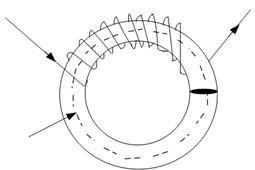## Magnetic Circuit Assignment Help

Assignment Help: >> ElectroMagnetism - Magnetic Circuit

Magnetic Circuit:

You know that electric circuit provides a path for electric current. Likewise, magnetic circuit provides a path for magnetic flux.

Example of a magnetic circuit is illustrated in Figure. Here we are considering an iron ring with a magnetic path of l meter, cross sectional area A m2 and a coil of N turns carrying I Amperes wound on it as illustrated in FigureFigure:  Magnetic Circuit

Now we will get acquainted with some significant terms associated to a magnetic circuit. These are magnetomotive force, flux density, magnetic field intensity, permeability, reluctance, etc.

Magnetic field is measured in terms of flux Φ which has unit weber (Wb). Flux density (B) is the flux per unit area, that menas. B = φ/ A. Magnetomotive Force (m.m.f.) is the force which drives flux through a magnetic circuit. Unit of m.m.f. is Ampere Turns (AT) and it is defined as the product of current and number of turns in a magnetic circuit. Thus, Magnetomotive force (m.m.f) = NI AT. Magnetomotive force per unit length of magnetic flux path is termed as magnetic field intensity (H) and it is described as

H = NI/ l AT/m .

So,

B = μ0 μr H = (μ0  μr NI )/ l  )Wb/m2

µ0 is the absolute permeability = 4π × 10- 7 henry/m and µr is the relative permeability of the medium.

Total Flux produced

φ= B × A =( μ0 μr A NI/ l) Wb

∴   φ= NI/( l/ μ0 μr A)    Wb#### Assured A++ Grade

Get guaranteed satisfaction & time on delivery in every assignment order you paid with us! We ensure premium quality solution document along with free turntin report!

All rights reserved! Copyrights ©2019-2020 ExpertsMind IT Educational Pvt Ltd